+
Finding the Sum of an Arithmetic Sequence
Solving Linear Equations and Inequalities
0
of 0 possible points

# Finding the Sum of an Arithmetic Sequence

Author: Sophia Tutorial
##### Description:

Calculate the sum of an arithmetic sequence.

(more)

Sophia’s self-paced online courses are a great way to save time and money as you earn credits eligible for transfer to many different colleges and universities.*

No credit card required

37 Sophia partners guarantee credit transfer.

299 Institutions have accepted or given pre-approval for credit transfer.

* The American Council on Education's College Credit Recommendation Service (ACE Credit®) has evaluated and recommended college credit for 33 of Sophia’s online courses. Many different colleges and universities consider ACE CREDIT recommendations in determining the applicability to their course and degree programs.

Tutorial
what's covered
1. Formula for the Sum of an Arithmetic Sequence
2. Finding the Sum when an is Given
3. Finding the Sum when an not Given

# 1. Formula for the Sum of an Arithmetic Sequence

Consider the following arithmetic sequence:

 {3, 8, 13, 18, 23, 28}

With only a few terms, it is easy enough to find the sum of all terms in this sequence. We can simply add the terms together to get a sum of 93. However, what if we had been given a sequence with 100 terms in it, and asked to find the sum? We still could add the terms concretely, but it would be inefficient. Let's take a look at an interesting shortcut.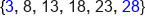Add the outermost terms: 3+28=31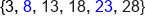Working our way in: 8+23=31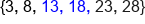Further in: 13+18=31

There is an interesting pattern when we add the first and last terms together, and then work our way to the center of the sequence. In each case, the sum is the same, 31. Due to this pattern, we know that the sum of all of the terms is going to be a multiple of 31. How many times should we multiply 31? We saw that we formed 3 pairs of 31, so the sum of all 6 terms in 93. To generalize this pattern, we need to think about the relationship between 6 terms and 3 pairs that sum to the same value. Since we were pairing terms, there are exactly half as many pairs as their are terms.

In general, then, to find the sum of an arithmetic sequence, we can add the first term and the n-th term, and then multiply that by the number of terms, n, divided by 2. Here is what our formula looks like:

formula
Sum of an Arithmetic Sequence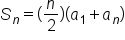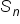The sum of n terms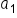The value of the 1st term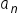The value of the nth term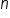The term

# 2. Finding the Sum when an is Given

Find the sum of the first 8 terms in the following sequence: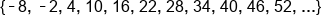Because we are summing the first through eighth terms of the sequence, we need to identify Term #1 and Term #8 to plug into our formula. We also need to know the value of n for our formula. Again, since we are summing 8 terms, n = 8. Now we can make all appropriate substitutions into our sum formula and solve: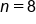,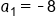,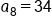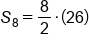Evaluate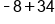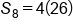Divide 8 by 2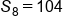Our Solution

# 3. Finding the Sum when an is not Given

In our final example, we are going to find the sum of a sequence when we aren't initially given the value of the nth term (or the last term in the sequence we are summing). The only difference between this example and the previous example is that we will need to calculate the value of the nth term ourselves. This isn't too difficult, because we have a formula for that as well.

formula
Arithmetic Sequence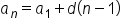The value of the nth termThe value of the 1st term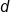Common differenceThe term

Find the sum of the first 100 terms of the following sequence: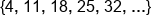In order to make use of our formula for the sum, we need to know the value of the first term and the value of the 100th term. We know the first term is 4, but we need to find the value of the 100th term. Let's use our formula to find the value of any term. This formula relies on knowing the common difference, or constant value that is added to get from one term to the next. We can quickly find the common difference by finding the difference between any two consecutive terms. We'll choose 18 and 25. The difference between these two numbers is 7. So 7 is d, the common difference in our formula: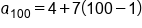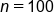,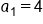,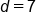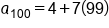Evaluate 100-1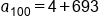Multiply 7 by 99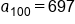Value of 100th terms

We aren't done yet. We just found the value of the 100th term that we need to be able to use the formula for finding the sum of the first 100 terms to the sequence. Now that we have all of the information we need, we can apply the sum formula: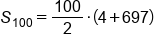,,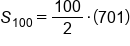Evaluate 4+697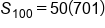Divide 100 by 2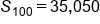Our Solution

This tells us that the sum of the first 100 terms in the arithmetic sequence is 35,050.

summary
When you want to find the sum of a sequence, it can be inefficient to actually sum the values of each term. Instead, we can use a formula for the sum of an arithmetic sequence. In this formula, n is the number of terms that you're summing, a1 is the first term, and an is the last or the nth term that you want to sum. When finding the sum when an is given, we just plug in the first and nth term into the formula. When finding the sum when an is not given, we don't have the nth term. We can determine an by using the formula for an arithmetic sequence, where a1 is the first term, d is the common difference, and n is the number of terms that you are summing.

Formulas to Know
Sum of an Arithmetic SequenceRating